# Functions

There are 366 different Starters of The Day, many to choose from. You will find below some starters on the topic of Functions. A lesson starter does not have to be on the same topic as the main part of the lesson or the topic of the previous lesson. It is often very useful to revise or explore other concepts by using a starter based on a totally different area of Mathematics.

Main Page

### Functions Starters: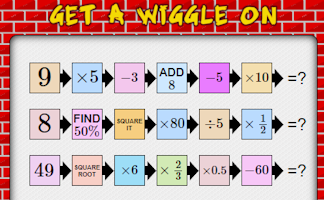A mixture of calculations to get your brain working at the start of a mathematics lesson.Change the numbers on the apples so that the number on the lemon is the given total.Arrange numbers at the bottom of the pyramid which will give the largest total at the top.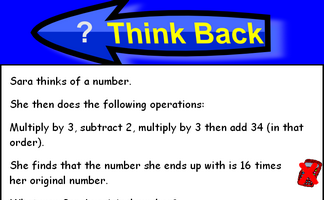A problem which can be answered by forming an algebraic equation then solving it.THOAN stands for 'Think of a number' and there are four randomly generated THOAN puzzles to solve.

## Exercises#### Coordinate Geometry Table

Fill in the empty cells of this table with information about lines, gradients and coordinates.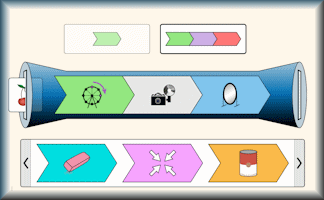#### Function Builder

An interactive function machine for patterns, numbers and equations.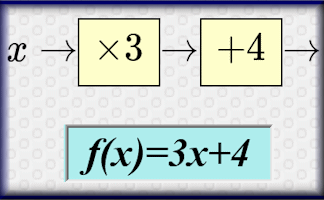#### Functions

An online exercise on function notation, inverse functions and composite functions.#### Graph Equation Pairs

Match the equation with its graph. Includes quadratics, cubics, reciprocals, exponential and the sine function.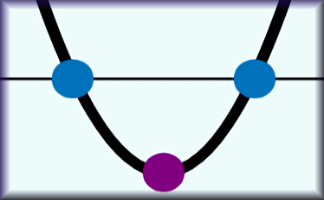Describe how changing the coefficients of a quadratic function changes the graph of the function#### Think of a Number

Ten students think of a number then perform various operations on that number. You have to find what the original numbers were.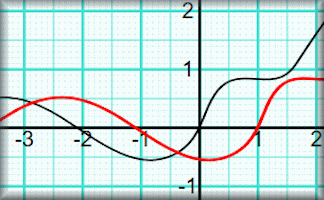#### Transformations of Functions

A visual aid showing how various transformations affect the graph of a function.

### Search

The activity you are looking for may have been classified in a different way from the way you were expecting. You can search the whole of Transum Maths by using the box below.

Have today's Starter of the Day as your default homepage. Copy the URL below then select
Tools > Internet Options (Internet Explorer) then paste the URL into the homepage field.

Set as your homepage (if you are using Internet Explorer)

Do you have any comments? It is always useful to receive feedback and helps make this free resource even more useful for those learning Mathematics anywhere in the world. Click here to enter your comments.For All: